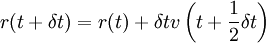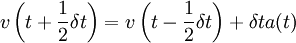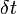# Verlet leap-frog algorithm$r(t + \delta t) = r (t) + \delta t v\left(t+ \frac{1}{2} \delta t\right)$$v \left(t+ \frac{1}{2} \delta t\right) = v\left(t - \frac{1}{2} \delta t\right) + \delta t a (t)$
where r is the position, v is the velocity, a is the acceleration and t is the time.$\delta t$ is known as the time step.